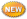← Back to X_TRADER® Help Library

### X_STUDY® Documentation

#### List of Technical Indicators

Technical Indicators are added to charts using the Technical Indicatorsmenu item on the Chart Area Context Menu.

Note: In the table below, Technical Indicators tagged as ‘New’ are only available with X_STUDY 7.8 and higher.

 Acceleration Moving Standard Bands (ABANDS)Accumulation/Distribution Average Convergence Divergence (MACD)Max Deviation (STDDEV)Stochastic (AD)Average (MAX)Money (STOCH)Stochastic Directional Movement (ADX)Adaptive Flow Index (MFI)Midpoint Fast (StochF)T3 Moving Average (AMA)Absolute (MIDPNT)Midprice (T3)Triple Price Oscillator (APO)Aroon (MIDPRI)Min Exponential Moving Average (TEMA)Triangular (AR)Aroon (MIN)MinMax Moving Average (TRIMA)Triple Oscillator (ARO)Average (MINMAX)Momentum Exponential Moving Average Oscillator (TRIX)Time True Range (ATR)Volume (MOM)Normalized Series Forecast (TSF)TT on the Ask (AVOL)Volume Average True Range (NATR)On Cumulative Vol Delta (TT CVD)Ultimate on the Bid and Ask (BAVOL)Bollinger Balance Volume (OBV)Price Oscillator (ULTOSC)Volume Band (BBANDS)Bar Channel (PC)PLOT At Price (VAP)Volume Value Area (BVA)Bid (PLT)Percent (VOLUME)Volume Volume (BVOL)Band Price Oscillator (PPO)Price Delta (Vol ∆)Volume Width (BW)Commodity Volume Trend (PVT)Rate Weighted Average Price (VWAP)Williams Channel Index (CCI)Chande of Change (ROC)Rate % R (WillR)Weighted Momentum Oscillator (CMO)Double of Change (ROC100)Rate Moving Average (WMA)Welles Exponential Moving Average (DEMA)Directional of Change (ROCP)Rate Wilder's Smoothing Average (WWS)Movement Indicators (DMI)Exponential of Change (ROCR)Relative (EMA)Fill Strength Indicator (RSI)Session Indicator (FILL)Ichimoku Volume (S_VOL)Parabolic (ICH)Keltner Sar (SAR)Session Channel (KC)Linear Cumulative Ask (SAVOL)Session Regression (LR)Linear Cumulative Bid (SBVOL)Simple Regression Angle (LRA)Linear Moving Average (SMA) Regression Intercept (LRI)Linear Regression Slope (LRM)